U.S. Geological Survey Data Series 420

# Seismic Profiles

#### Home | Acronyms | Contents | Navigation | Profiles | Logs | Metadata | Software

Below are thumbnail images of each line with links to full-size printable and interactive seismic reflection profiles. The printable profiles are GIF images that were filtered and gained using Seismic Unix software. These images are stored in the PRINTABLE folder. Refer to the Software page for details about the processing and examples of the processing scripts. The processed SEG-Y data were exported to CTI SonarWeb software to produce interactive profiles that allow the user to obtain a geographic location and depth from the profile for a cursor position. This information is displayed in the status bar of the browser. The interactive profiles, which are stored in the INTERACTIVE folder, have been corrected for the offset between the shotpoint and GPS antenna, see figure 1 and figure 2.

The Navigation page has links to the trackline maps, which illustrate the locations of the interactive and printable profiles presented below.

Table 2 lists trackline statistics for 08LCA03.

NOTE: The only supported Web browsers that properly display all features of the interactive profiles are Internet Explorer 6 or greater for Windows and Firefox 1.5 or greater for all platforms. Use of other browsers may result in spurious or no information given in the status window. Firefox may be downloaded at http://www.mozilla.org/products/firefox.

NOTE: No data were collected for trackline 08b08; therefore, no interactive or printable profile or links were created for this line.

Browser setup for viewing interactive profiles:
For all browsers, the status bar and JavaScript must be enabled. Firefox also requires "Change status bar text" to be checked under Advanced JavaScript Options.

If using Internet Explorer 7, Active Content/ActiveX controls must be disabled. Instructions for doing this can be found by clicking on the "details and setup" link on the interactive profile pages.

Line: 08b01  3821 traces:
Start of line:   08b01     SHOT=1 day=134 hour=17  minute=48  sec=19
End of line:   08b01  SHOT=3821 day=134 hour=18  minute=20  sec=47
INTERACTIVE | PRINTABLE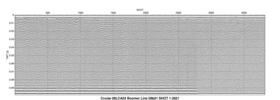Line: 08b02  2453 traces:
Start of line:   08b02     SHOT=1 day=134 hour=18  minute=25  sec=37
End of line:   08b02  SHOT=2453 day=134 hour=18  minute=46  sec=28
INTERACTIVE | PRINTABLE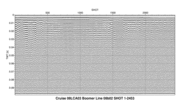Line: 08b03  3359 traces:
Start of line:   08b03     SHOT=1 day=134 hour=18  minute=46  sec=47
End of line:   08b03  SHOT=3359 day=134 hour=19  minute=15  sec=19
INTERACTIVE | PRINTABLE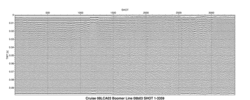Line: 08b04  2176 traces:
Start of line:   08b04     SHOT=1 day=134 hour=19  minute=25  sec=37
End of line:   08b04  SHOT=2176 day=134 hour=19  minute=44   sec=6
INTERACTIVE | PRINTABLE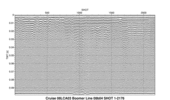Line: 08b05  2442 traces:
Start of line:   08b05     SHOT=1 day=134 hour=19  minute=44  sec=48
End of line:   08b05  SHOT=2442 day=134 hour=20   minute=5  sec=33
INTERACTIVE | PRINTABLE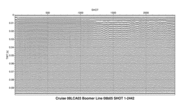Line: 08b06  3174 traces:
Start of line:   08b06     SHOT=1 day=134 hour=20   minute=6  sec=39
End of line:   08b06  SHOT=3174 day=134 hour=20  minute=33  sec=37
INTERACTIVE | PRINTABLE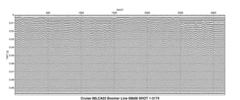Line: 08b07  2688 traces:
Start of line:   08b07     SHOT=1 day=134 hour=20  minute=34  sec=58
End of line:   08b07  SHOT=2688 day=134 hour=20  minute=57  sec=49
INTERACTIVE | PRINTABLE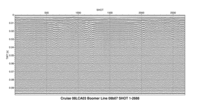Line: 08b09  5671 traces:
Start of line:   08b09     SHOT=1   day=0 hour=14  minute=17  sec=30
End of line:   08b09  SHOT=5671   day=0 hour=15   minute=4  sec=46
INTERACTIVE | PRINTABLE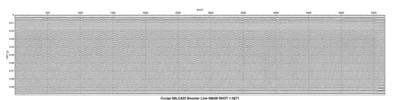Line: 08b10  3487 traces:
Start of line:   08b10     SHOT=1   day=0 hour=15   minute=4  sec=46
End of line:   08b10  SHOT=3487   day=0 hour=15  minute=33  sec=50
INTERACTIVE | PRINTABLE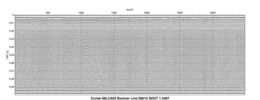Line: 08b11  1070 traces:
Start of line:   08b11     SHOT=1   day=0 hour=15  minute=33  sec=50
End of line:   08b11  SHOT=1070   day=0 hour=15  minute=42  sec=44
INTERACTIVE | PRINTABLE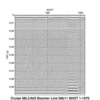Line: 08b12   513 traces:
Start of line:   08b12     SHOT=1   day=0 hour=15  minute=42  sec=44
End of line:   08b12     SHOT=3   day=0 hour=15  minute=47   sec=8
INTERACTIVE | PRINTABLE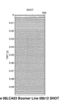Line: 08b13   569 traces:
Start of line:   08b13     SHOT=1   day=0 hour=15  minute=49  sec=22
End of line:   08b13   SHOT=569   day=0 hour=15  minute=54   sec=6
INTERACTIVE | PRINTABLE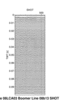Line: 08b14  1812 traces:
Start of line:   08b14     SHOT=1   day=0 hour=16  minute=10  sec=52
End of line:   08b14  SHOT=1812   day=0 hour=16  minute=25  sec=58
INTERACTIVE | PRINTABLE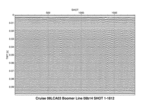Line: 08b15  1234 traces:
Start of line:   08b15     SHOT=1   day=0 hour=16  minute=25  sec=58
End of line:   08b15  SHOT=1234   day=0 hour=16  minute=36  sec=14
INTERACTIVE | PRINTABLE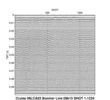Line: 08b16  1405 traces:
Start of line:   08b16     SHOT=1   day=0 hour=16  minute=37  sec=36
End of line:   08b16  SHOT=1405   day=0 hour=16  minute=49  sec=18
INTERACTIVE | PRINTABLE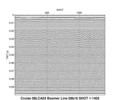Line: 08b17  1578 traces:
Start of line:   08b17     SHOT=1   day=0 hour=16  minute=49  sec=18
End of line:   08b17  SHOT=1578   day=0 hour=17   minute=2  sec=26
INTERACTIVE | PRINTABLE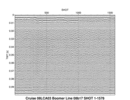Line: 08b18  2256 traces:
Start of line:   08b18     SHOT=1   day=0 hour=17   minute=2  sec=26
End of line:   08b18  SHOT=2256   day=0 hour=17  minute=21  sec=14
INTERACTIVE | PRINTABLE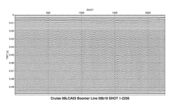Line: 08b19  3555 traces:
Start of line:   08b19     SHOT=1   day=0 hour=17  minute=21  sec=14
End of line:   08b19  SHOT=3555   day=0 hour=17  minute=50  sec=52
INTERACTIVE | PRINTABLE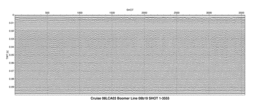Line: 08c01  3642 traces:
Start of line:   08c01     SHOT=1 day=134 hour=14  minute=57   sec=0
End of Line:   08c01  SHOT=3642 day=134 hour=15  minute=12  sec=30
INTERACTIVE | PRINTABLE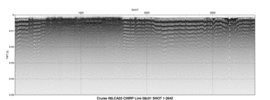Line: 08c02  5600 traces:
Start of line:   08c02     SHOT=1 day=134 hour=15  minute=13  sec=38
End of Line:   08c02  SHOT=5600 day=134 hour=15  minute=37  sec=14
INTERACTIVE | PRINTABLE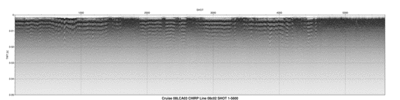#### Home | Acronyms | Contents | Navigation | Profiles | Logs | Metadata | Software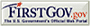U.S. Department of the Interior | U.S. Geological Survey
URL: pubs.usgs.gov/ds/420/html/profiles.html
Page Contact Information: Feedback